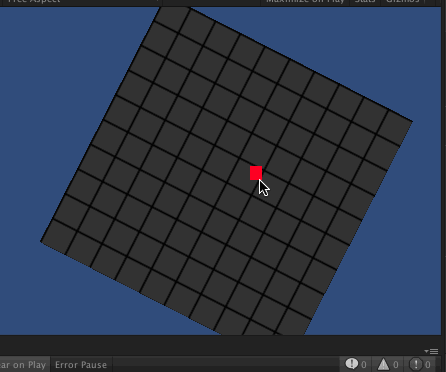# Snap object to grid

hi guys.

i’ve made a grid by creating a plane and set grid texture on it,besides I had a script for drag-and-drop object.

Now, i trying to create a script that when i release the mouse, the object i’ve been dragging snaps to the line of grid (in the picture shown below, the red is object). Can anyone help me? thanks in advance !``````//Drag-and-Drop script

using UnityEngine;

using System.Collections;

public class MovePoint2 : MonoBehaviour
{
private Vector3 screenPoint;
private Vector3 offset;

void OnMouseDown()
{
screenPoint = Camera.main.WorldToScreenPoint(gameObject.transform.position);

offset = gameObject.transform.position - Camera.main.ScreenToWorldPoint(new Vector3(Input.mousePosition.x, Input.mousePosition.y, screenPoint.z));

Screen.showCursor = false;

}

void OnMouseDrag()
{
Vector3 curScreenPoint = new Vector3(Input.mousePosition.x, Input.mousePosition.y, screenPoint.z);

Vector3 curPosition = Camera.main.ScreenToWorldPoint(curScreenPoint) + offset;

transform.position = curPosition;

}

void OnMouseUp()
{
Screen.showCursor = true;
}
}
``````

Here’s a function you just need to call to snap to your grid object.
The grid width/height is determined from the material’s tiling, (my texture is just an edge, then repeated to make a grid)

Then we manipulate the position relative to the grid (a quad in my case) so it’s irrelevant how much it’s scaled, and even works when rotated!``````Vector3 SnapToGrid(Vector3 Position)
{
GameObject grid = GameObject.Find ("grid");
if (! grid)
return Position;

//	get grid size from the texture tiling
Vector2 GridSize = grid.renderer.material.mainTextureScale;

//	get position on the quad from -0.5...0.5 (regardless of scale)
Vector3 gridPosition = grid.transform.InverseTransformPoint( Position );
//	scale up to a number on the grid, round the number to a whole number, then put back to local size
gridPosition.x = Mathf.Round( gridPosition.x * GridSize.x ) / GridSize.x;
gridPosition.y = Mathf.Round( gridPosition.y * GridSize.y ) / GridSize.y;

//	don't go out of bounds
gridPosition.x = Mathf.Min ( 0.5f, Mathf.Max (-0.5f, gridPosition.x) );
gridPosition.y = Mathf.Min ( 0.5f, Mathf.Max (-0.5f, gridPosition.y) );

Position = grid.transform.TransformPoint( gridPosition );
return Position;
}
``````

Then change OnMouseDrag to include this to snap-as-you-drag

``````transform.position = SnapToGrid(curPosition);
``````

or when you let go, in OnMouseUp…

``````transform.position = SnapToGrid(transform.position);
``````

My example is just a square, but you just need to change the square so the center is the top of your line, or make a small adjustment to the final Postion = line

[26022-snaptogrid699158.zip|26022]